# NERD force field

Jump to: navigation, search

The NERD force field for alkanes was developed by Nath, Escobedo, and de Pablo (Ref. 1) (with the addition of the word Revised one arrives at NERD, see reference 15 within Ref. 1. The amusing nature of the name in English was far from lost on the authors). The NERD parameters show a certain similitude to other alkane parameter sets, such as those of López Rodríguez and co-workers (Refs. 2-4) or those of Smit, Karaborni, and Siepmann (SKS) (Refs. 5-7).

## Parameters

In the NERD model the non-bonded intermolecular pair potential is that of the Lennard-Jones model with the following parameters (Ref. 1 Table I):

 Molecule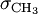$\sigma_{\mathrm {CH}_3}$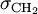$\sigma_{\mathrm {CH}_2}$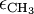$\epsilon_{\mathrm {CH}_3}$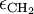$\epsilon_{\mathrm {CH}_2}$ ethane 3.825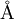$\mathrm{\AA}$ 3.93$\mathrm{\AA}$ 100.6 K propane 3.857$\mathrm{\AA}$ 3.93$\mathrm{\AA}$ 102.6 K 45.8 K butane and longer 3.91$\mathrm{\AA}$ 3.93$\mathrm{\AA}$ 104.0 K 45.8 K